Test: Chemical Equilibrium - 2

# Test: Chemical Equilibrium - 2

Test Description

## 25 Questions MCQ Test Physical Chemistry | Test: Chemical Equilibrium - 2

Test: Chemical Equilibrium - 2 for Chemistry 2022 is part of Physical Chemistry preparation. The Test: Chemical Equilibrium - 2 questions and answers have been prepared according to the Chemistry exam syllabus.The Test: Chemical Equilibrium - 2 MCQs are made for Chemistry 2022 Exam. Find important definitions, questions, notes, meanings, examples, exercises, MCQs and online tests for Test: Chemical Equilibrium - 2 below.
Solutions of Test: Chemical Equilibrium - 2 questions in English are available as part of our Physical Chemistry for Chemistry & Test: Chemical Equilibrium - 2 solutions in Hindi for Physical Chemistry course. Download more important topics, notes, lectures and mock test series for Chemistry Exam by signing up for free. Attempt Test: Chemical Equilibrium - 2 | 25 questions in 90 minutes | Mock test for Chemistry preparation | Free important questions MCQ to study Physical Chemistry for Chemistry Exam | Download free PDF with solutions
 1 Crore+ students have signed up on EduRev. Have you?
Test: Chemical Equilibrium - 2 - Question 1

### An example of a reversible reaction is:

Test: Chemical Equilibrium - 2 - Question 2

###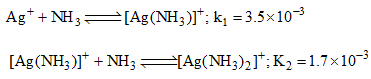Then the formation constant of [Ag(NH3)2]+ is:

Test: Chemical Equilibrium - 2 - Question 3

### Does Le-Chatelier’s principle predict a change of equilibrium concentrations for the following reaction if the gas mixture is compressed: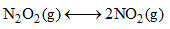Detailed Solution for Test: Chemical Equilibrium - 2 - Question 3

N2​O4​(g)⇌2NO2​(g)

One mole of N2​O4​ gives 2 moles of NO2​ gas. The pressure of the gas increases. Upon compressing the gaseous mixture, there is a change in an equilibrium concentration & backward reaction is favoured. This is according to Le Chatlier's Principle

Test: Chemical Equilibrium - 2 - Question 4

The plot given below shows the relation between (1/T) and log(s), where S is the solubility of an electrolyte AB and T is the temperature in Kelvin. What conclusion can be drawn from the plot: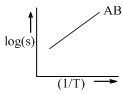Test: Chemical Equilibrium - 2 - Question 5

A mixture of 2 mole each of helium and an unknown gas (normal boiling point = 0°C) is kept in a 22.4 L flask. If the flask is cooled to 0.1°C, the resultant pressure (in atm) inside the flask is:

Test: Chemical Equilibrium - 2 - Question 6

For the complete oxidation of 100 g of Cyclohexanol to cyclohexanon, the quantity of CrO3 required is (assuming 100% chemical yield) [Atomic wt. of Cr = 52)

Detailed Solution for Test: Chemical Equilibrium - 2 - Question 6

As we know that, Cr = 52,
O3 = 16*3 = 48
CrO3 = 52 = 48
= 100 gm

Test: Chemical Equilibrium - 2 - Question 7

For the reaction: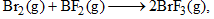the equilibrium constant at 2000 K and 1.0 bar is 5.25. When the pressure is increased by 8-fold, the equilibrium constant:

Test: Chemical Equilibrium - 2 - Question 8

In a chemical reaction,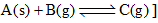The total pressure at equilibrium is 6 atm. The value of equilibrium constant is:

Test: Chemical Equilibrium - 2 - Question 9

If the equilibrium constants for the reactions 1 and 2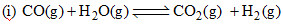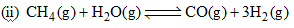Are K1 and K2, the equilibrium constant for the reaction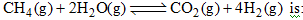Test: Chemical Equilibrium - 2 - Question 10

Consider the reaction,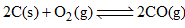at equilibrium,

The equilibrium can be shifted towards the forward direction by:

Test: Chemical Equilibrium - 2 - Question 11

When a reversible reaction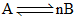is followed with time with initial concentration of A being 0.6 mol. L–1, the following graph is obtained: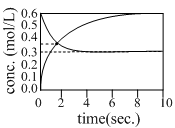The equilibrium constant K, for the above reaction is:

Test: Chemical Equilibrium - 2 - Question 12

If the equilibrium reaction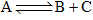is heated, it is observed that the concentration of A increases. Then,

Test: Chemical Equilibrium - 2 - Question 13

Consider the reaction: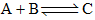The unit of the thermodynamic equilibrium constant for the reaction is:

Detailed Solution for Test: Chemical Equilibrium - 2 - Question 13

An equilibrium constant is defined to be equal to the ratio of the forward and backward reaction rate constants. This particular concentration quotient, , has the dimension of concentration, but the thermodynamic equilibrium constant, K, is always dimensionless.

Test: Chemical Equilibrium - 2 - Question 14

For the reaction H2(g)+I2(g)⇌2HI (g) at 721 K
value of equilibrium constant is 50, when molar concentration of both hydrogen and iodine is 0.5 M at equilibrium value of Kp under the same conditions will be

Detailed Solution for Test: Chemical Equilibrium - 2 - Question 14

The equilibrium reaction is H2​(g)+I2​(g)⇔2HI(g).
The relationship between Kp​ and Kc​ is Kp​=Kc​(RT)Δn.
For the equilibrium reaction, Δn=2−(1+1)=0.
Hence, Kp​=Kc​=50.

*Answer can only contain numeric values
Test: Chemical Equilibrium - 2 - Question 15

A 2 L vessel containing 2g of H2 gas at 27°C is connected to a 2L vessel containing 176 g of CO2 gas at 27°C. Assuming ideal behaviour of H2 and CO2, the partial pressure of H2 at equilibrium is………bar.

Detailed Solution for Test: Chemical Equilibrium - 2 - Question 15

H2 + CO2 - mixture of non-reacting gases
2g H2 = 1 mol
176g CO2 = 4 mol
Total no. of moles = 5
Total volume = 2+2 = 4L
Temperature = 27+273 = 300K
Total pressure, P=nRT/V=(5*0.083*300/4)= 31.125 bar
According to Dalton's law, p1 = P*(x1)
Mole fraction of H2=1/(1+4)=1/5
Therefore, p(H2)= 31.125*0.2 = 6.225 bar

Test: Chemical Equilibrium - 2 - Question 16

The relationship between the equilibrium constant K1 for the reaction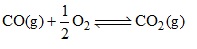And the equilibrium constant K2 for the reaction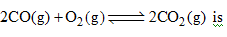Test: Chemical Equilibrium - 2 - Question 17

The hydrolysis constant (Kh) of NH4Cl is 5.6×10–10. The concentration of H3O+ in a 0.1 M solution of NH4Cl at equilibrium is:

Test: Chemical Equilibrium - 2 - Question 18

The equilibrium constant Kc for the following reaction at 842°C is 7.90 × 10–3. What is Kp at same temperature: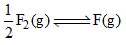Test: Chemical Equilibrium - 2 - Question 19

For the equilibrium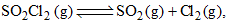what is the temperature at which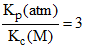Test: Chemical Equilibrium - 2 - Question 20

At a certain temperature, the following reactions have the equilibrium constants as shown below:

S(s) + O2(g) <=> SO2(g); Kc1 = 5 X 1052

2S(s) + 3O2(g) <=> 2SO3(g) ; Kc2 = 1029

What is the equilibrium constant, Kc​ for the reaction at the same temperature?

2SO2(g) + O2(g) <=> 2SO3(g)

Detailed Solution for Test: Chemical Equilibrium - 2 - Question 20

Equilibrium reactions depend upon the reaction equations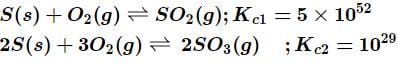The Kc​ for the reaction is calculated as,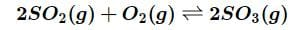By the equation,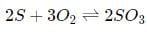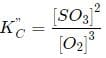By reversing the first equation and doubling the coefficient Kc

(KC,​)−1

Multiply both side of the equation  by K,

2.5×1076

Test: Chemical Equilibrium - 2 - Question 21

Consider the following gaseous equilibria given below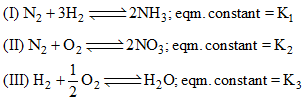The equilibrium constant for the reaction,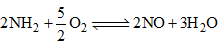in terms of K1, K2 and K3 will be

Detailed Solution for Test: Chemical Equilibrium - 2 - Question 21

Explanation : K1 = [NH3]2/[N2] [H2]3

K2 = [NO]2/[N2] [O2]3

K3 = [H2O]/[O2]1/2 [H2]

For the reaction :

K = {[NO]2 [H2O]3}/{[NH3]2 [O2]3/2}

= (K2 K33)/K1

Test: Chemical Equilibrium - 2 - Question 22

9.2 grams of N2O4(g) is taken in a closed on litre vessel and heated till the following equilibrium is reached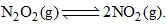At equilibrium, 50% N2O4(g) is dissociated. What is the equilibrium constant (in mol litre–1) (molecular weight of N2O4 = 92)

Test: Chemical Equilibrium - 2 - Question 23

In a chemical reaction: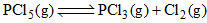xenon gas is added at constant volume. The equilibrium:

Test: Chemical Equilibrium - 2 - Question 24

The equilibrium constants for the reactions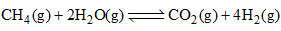and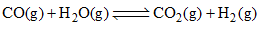are K1 and K2, respectively. The equilibrium constant for the reaction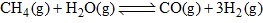is

Test: Chemical Equilibrium - 2 - Question 25

Correct statement on the effect of addition of aq. HCl on the equilibrium is: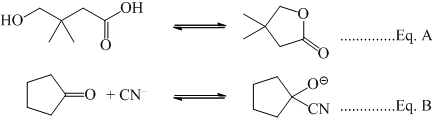## Physical Chemistry

83 videos|106 docs|31 tests
 Use Code STAYHOME200 and get INR 200 additional OFF Use Coupon Code
Information about Test: Chemical Equilibrium - 2 Page
In this test you can find the Exam questions for Test: Chemical Equilibrium - 2 solved & explained in the simplest way possible. Besides giving Questions and answers for Test: Chemical Equilibrium - 2, EduRev gives you an ample number of Online tests for practice

## Physical Chemistry

83 videos|106 docs|31 tests